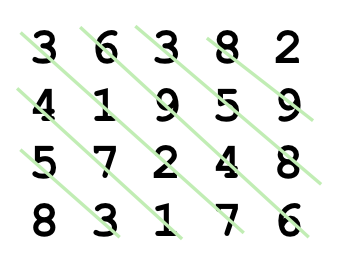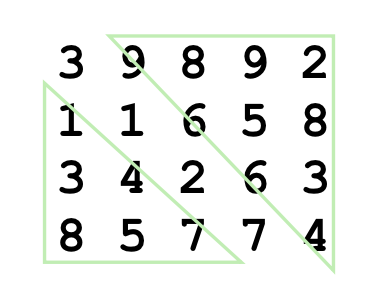XDAYS

:

HOUR

:

MINS

:

SEC

Copied to Clipboard
Sort a 2D vector diagonally
Medium Accuracy: 65.01% Submissions: 4637 Points: 4

Given an NxM 2D matrix, rearrange such that
Each diagonal in the lower left triangle of the rectangular grid is sorted in ascending order.
Each diagonal in the upper right triangle of the rectangular grid is sorted in descending order.
The major diagonal in the grid starting from the top-left corner is not rearranged.

Example 1:

Input:
N = 4, M = 5
matrix = {{3 6 3 8 2},
{4 1 9 5 9},
{5 7 2 4 8},
{8 3 1 7 6}}
Output:
3 9 8 9 2
1 1 6 5 8
3 4 2 6 3
8 5 7 7 4
Explanation:
Before:After:You don't need to read input or print anything. Your task is to complete the function diagonalSort() which takes the matrix, n and m as input parameter and rearranges the elements of the matrix.

Expected Time Complexity: O(NxM)
Expected Auxiliary Space: O(N+M)

Constraints:
1 <= N,M <= 10, 1<=N*M<=105
1 <= matrix[i] <= 103

We are replacing the old Disqus forum with the new Discussions section given below.

Editorial

We strongly recommend solving this problem on your own before viewing its editorial. Do you still want to view the editorial?

My Submissions: